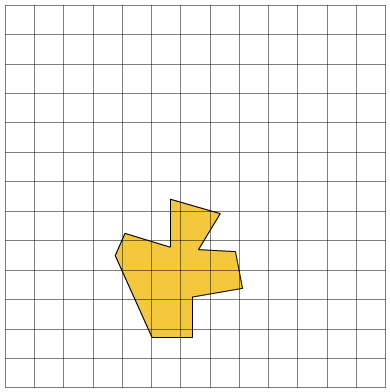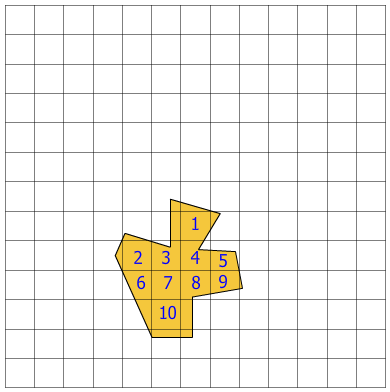# Area of Irregular Figures on Graphs

Rate 0 stars Common Core
Quiz size:
Message preview:
Someone you know has shared quiz with you:

To play this quiz, click on the link below:

https://www.turtlediary.com/quiz/area-of-irregular-figures-on-graphs.html

Hope you have a good experience with this site and recommend to your friends too.

Login to rate activities and track progress.
Login to rate activities and track progress.

To find the area of complex figures, count only completely filled squares or squares more than half-filled.

For example, let's find the area of the figure given below.To find the area of this figure, count the completely filled and more than half-filled squares.We observe that the number of completely filled and more than half-filled squares is 10.

So, the area of the given figure is 10 square units.

ds

A B C D E F G H I J K L M N O P Q R S T U V W X Y Z

### Help

##### Remember :

The smallest number is the one that comes first while counting.

##### Solution :

To arrange the given numbers in order from smallest to greatest, find the smallest number among all the given numbers.

21,27,23

21 is the smallest number.